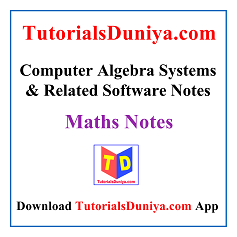## Computer Algebra Systems & Related Software Notes PDF

Date: 27th Jan 2023

In these “Computer Algebra Systems & Related Software Notes pdf”, we will study the usage of computer algebra systems (/Mathematica/MATLAB/Maxima/Maple) and the statistical software R. The basic emphasis is on plotting and working with matrices using CAS. Data entry and summary commands will be studied in R. Graphical representation of data shall also be explored.

We have provided multiple complete Computer Algebra Systems & Related Software Notes PDF for any university student of BCA, MCA, B.Sc, B.Tech CSE, M.Tech branch to enhance more knowledge about the subject and to score better marks in the exam. Students can easily make use of all these Computer Algebra Systems & Related Software Notes PDF by downloading them.

Topics in our Computer Algebra Systems & Related Software Notes PDF

The topics we will cover in these Computer Algebra Systems & Related Software Notes PDF will be taken from the following list:

Introduction to CAS and Applications: Computer Algebra System (CAS), Use of a CAS as a calculator, Computing and plotting functions in 2D, Plotting functions of two variables using Plot3D and ContourPlot, Plotting parametric curves surfaces, Customizing plots, Animating plots, Producing tables of values, working with piecewise-defined functions, Combining graphics.

Working with Matrices: Simple programming in a CAS, Working with matrices, Performing Gauss elimination, operations (transpose, determinant, inverse), Minors and cofactors, Working with large matrices, Solving system of linear equations, Rank and nullity of a matrix, Eigenvalue, eigenvector and diagonalization.

R – The Statistical Programming Language: R as a calculator, Explore data and relationships in R. Reading and getting data into R: Combine and scan commands, Types, and structure of data items with their properties, Manipulating vectors, Data frames, Matrices, and lists, Viewing objects within objects, Constructing data objects and conversions.

Data Analysis with R: Summary commands: Summary statistics for vectors, Data frames, Matrices and lists, Summary tables, Stem and leaf plot, Histograms, Plotting in R: Box-whisker plots, Scatter plots, Pairs plots, Line charts, Pie charts, Cleveland dot charts and bar charts, Copy and save graphics to other applications.Computer Algebra Systems & Related Software Notes
Source: researchgate.netComputer Algebra Systems & Related Software Notes
Source: inf.ed.ac.ukComputer Algebra System pdf Notes
Source: math.wpi.eduComputer Algebra Systems & Related Software Notes
Source: csd.uwo.caComputer Algebra System pdf Notes
Source: math.usm.eduComputer Algebra Systems & Related Software Notes
Source: maplesoft.comComputer Algebra System pdf Notes
Source: math.uic.eduComputer Algebra Systems & Related Software Notes
Source: springer.com

### Computer Algebra Systems & Related Software Reference Books List

We have listed the best Computer Algebra Systems & Related Software Reference Books that can help in your Computer Algebra Systems & Related Software exam preparation:

• abc
• abc

### Maths Notes PDF

##### Software Engineering Projects PDF with Documentation ReportIn this article, you will get the list of Best Software Engineering Projects pdf with Documentation. These software engineering mini projects pdf will help you tremendously in preparation for your own Software Engineering Project report.

URL: https://www.tutorialsduniya.com/software-engineering-projects-pdf/

Author: Delhi University

Editor's Rating:
5